This document is part of the HTML publication "An Introduction to the Imperative Part of C++"

The original version was produced by Rob Miller at Imperial College London, September 1996.

Version 1.1 (modified by David Clark at Imperial College London, September 1997)

Version 1.2 (modified by Bob White at Imperial College London, September 1998)

Version 1.3, 1.4, 2.0, ..., 2.21 (modified by William Knottenbelt at Imperial College London, September 1999-September 2022)

# 8. Recursion

## 8.1 The Basic Idea

We have already seen how, in a well designed C++ program, many function definitions include calls to other functions (for example, in the last lecture the definition of "assign_list(...)" included a call to "assign_new_node(...)"). A function is recursive (or has a recursive definition) if the definition includes a call to itself.

Recursion is a familiar idea in mathematics and logic. For example, the natural numbers themselves are usually defined recursively. Very roughly speaking, the definition is:

• 0 is a natural number.
• if n is a natural number then s(n) (i.e. n+1) is a natural number, where s is the "successor function".

In this context, the notion of recursion is clearly related to the notion of mathematical induction. Notice also that the above definition includes a non-recursive part or base case (the statement that 0 is a natural number).

Another familiar mathematical example of a recursive function is the factorial function "!". Its definition is:

• 0! = 1
• for all n > 0, n! = nx(n-1)!

Thus, by repeatedly using the definition, we can work out that

```
6! = 6x5! = 6x5x4! = 6x5x4x3! = 6x5x4x3x2! = 6x5x4x3x2x1! = 6x5x4x3x2x1x1 = 720

```

Again, notice that the definition of "!" includes both a base case (the definition of 0!) and a recursive part.

## 8.2 A Simple Example

The following program includes a call to the recursively defined function "print_backwards()", which inputs a series of characters from the keyboard, terminated with a full-stop character, and then prints them backwards on the screen.

``` 	#include<iostream>
using namespace std;

void print_backwards();

int main()
{
print_backwards();
cout << "\n";

return 0;
}

void print_backwards()
{
char character;

cout << "Enter a character ('.' to end program): ";
cin >> character;
if (character != '.')
{
print_backwards();
cout << character;
}
}
```

A typical input/output session is:

```	Enter a character ('.' to end program): H
Enter a character ('.' to end program): i
Enter a character ('.' to end program): .
iH
```

We will examine how this function works in more detail in the next section. But notice that the recursive call to "print_backwards()" (within its own definition) is embedded in an "if" statement. In general, recursive definitions must always use some sort of branch statement with at least one non-recursive branch, which acts as the base case of the definition. Otherwise they will "loop forever". In Program 8.2.1 the base case is in the implicit "else" part of the "if" statement. We could have written the function as follows:

```	void print_backwards()
{
char character;

cout << "Enter a character ('.' to end program): ";
cin >> character;
if (character != '.')
{
print_backwards();
cout << character;
}
else
{
;
}
}
```

## 8.3 The Mechanics of a Recursive Call

It is easy to see why Program 8.2.1 works with the aid of a few diagrams. When the main program executes, it begins with a call to "print_backwards()". At this point space is set aside in the computer's memory to execute this call (and in other cases in which to make copies of the value parameters). This space is represented as a box in Figure 8.3.1a: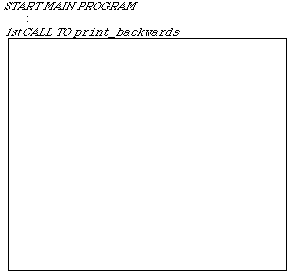Figure 8.3.1a

The internal execution of this call begins with a character input, and then a second call to "print_backwards()" (at this point, nothing has been output to the screen). Again, space is set aside for this second call: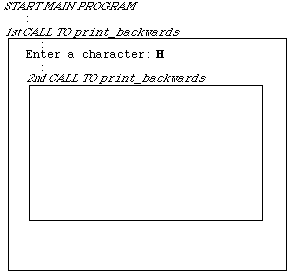Figure 8.3.1b

The process repeats, but inside the third call to "print_backwards()" a full-stop character is input, thus allowing the third call to terminate with no further function calls: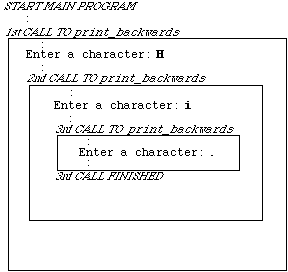Figure 8.3.1c

This allows the second call to "print_backwards()" to terminate by outputting an "i" character, which in turn allows the first call to terminate by outputting an "H" character: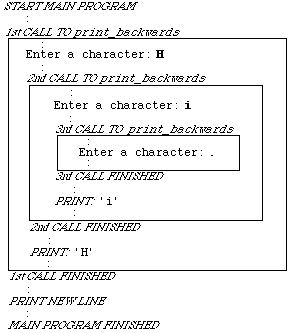Figure 8.3.1d

Technically speaking, C++ arranges the memory spaces needed for each function call in a stack. The memory area for each new call is placed on the top of the stack, and then taken off again when the execution of the call is completed. In the example above, the stack goes through the following sequence: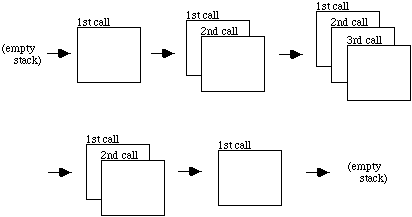Figure 8.3.2

C++ uses this stacking principle for all nested function calls - not just for recursively defined functions. A stack is an example of a "last in/first out" structure (as opposed to, for example, a queue, which is a "first in/first out" structure).

## 8.4 Three More Examples

Below are three more examples of recursive functions. We have already seen a function to calculate the factorial of a positive integer (Lecture 3, Program 3.3.1). Here's an alternative, recursive definition:

```	int factorial(int number)
{
if (number < 0)
{
cout << "\nError - negative argument to factorial\n";
exit(1);
}
else if (number == 0)
return 1;
else
return (number * factorial(number - 1));
}
```

Fragment of Program 8.4.1

As a second example, here's a function which raises its first argument (of type "float") to the power of its second (non-negative integer) argument:

```	float raised_to_power(float number, int power)
{
if (power < 0)
{
cout << "\nError - can't raise to a negative power\n";
exit(1);
}
else if (power == 0)
return (1.0);
else
return (number * raised_to_power(number, power - 1));
}
```

Fragment of Program 8.4.2

In both cases, care has been taken that a call to the function will not cause an "infinite loop" - i.e. that the arguments to the functions will either cause the program to exit with an error message, or are such that the series of recursive calls will eventually terminate with a base case.

The third example recursive function sums the first n elements of an integer array "a[]".

```	int sum_of(int a[], int n)
{
if (n < 1 || n > MAXIMUM_NO_OF_ELEMENTS)
{
cout << "\nError - can only sum 1 to ";
cout << MAXIMUM_NO_OF_ELEMENTS << " elements\n";
exit(1);
}
else if (n == 1)
return a;
else
return (a[n-1] + sum_of(a,n-1));
}
```

Fragment of Program 8.4.3

## 8.5 Recursion and Iteration

From a purely mechanical point of view, recursion is not absolutely necessary, since any function that can be defined recursively can also be defined iteratively, i.e. defined using "for", "while" and "do...while" loops. So whether a function is defined recursively or iteratively is to some extent a matter of taste. Here are two of the recursive functions above, re-defined iteratively:

```	void print_backwards()
{
char chars[MAX_ARRAY_LENGTH];
int no_of_chars_input = 0;

do {
cout << "Enter a character ('.' to end program): ";
cin >> chars[no_of_chars_input];
no_of_chars_input++;
}
while (chars[no_of_chars_input - 1] != '.'
&& no_of_chars_input < MAX_ARRAY_LENGTH);

for (int count = no_of_chars_input - 2 ; count >=0 ; count--)
cout << chars[count];
}
```

Fragment of Program 8.2.1b

```	int factorial(int number)
{
int product = 1;

if (number < 0)
{
cout << "\nError - negative argument to factorial\n";
exit(1);
}
else if (number == 0)
return 1;
else
{
for ( ; number > 0 ; number--)
product *= number;

return product;
}
}
```

Fragment of Program 8.4.1b

It is a matter of debate whether, for a particular function, a recursive definition is clearer than an iterative one. Usually, an iterative definition will include more local variable declarations - for example, the array "chars[MAX_ARRAY_LENGTH]" in the first example above, and the integer variable "product" in the second example. In other words, temporary memory allocation is made explicit in the iterative versions of the functions by declaring variables, whereas it is implicit in the recursive definitions (C++ is implicitly asked to manipulate the stack by use of recursive calls).

Because of extra stack manipulation, recursive versions of functions often run slower and use more memory than their iterative counterparts. But this is not always the case, and recursion can sometimes make code easier to understand.

## 8.6 Recursive Data Structures

Recursive function definitions are often particularly useful when the program is manipulating recursive data structures. We have already seen one definition of a recursive data structure - the definition of a node in a linked list is given in terms of itself:

```	struct Node
{
char word[MAX_WORD_LENGTH];
Node *ptr_to_next_node;
};
```

Later in the course you will study other recursive data structures in more detail, and see how associated recursive function definitions behave in these contexts.

## 8.7 Quick Sort - A Recursive Procedure for Sorting

We will end this lecture by briefly looking at a standard recursive procedure for sorting. Quick sort is a recursively defined procedure for rearranging the values stored in an array in ascending or descending order.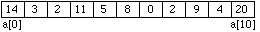Figure 8.7.1

The idea is to use a process which separates the list into two parts, using a distinguished value in the list called a pivot. At the end of the process, one part will contain only values less than or equal to the pivot, and the other will contain only values greater than or equal to the pivot. So, if we pick 8 as the pivot, at the end of the process we will end up with something like: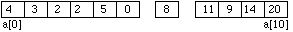Figure 8.7.2

We can then reapply exactly the same process to the left-hand and right-hand parts separately. This re- application of the same procedure leads to a recursive definition.

The detail of the rearranging procedure is as follows. The index of the pivot value is chosen simply by evaluating

```	(first + last) / 2
```

where "first" and "last" are the indices of the initial and final elements in the array representing the list. We then identify a "left_arrow" and a "right_arrow" on the far left and the far right respectively. This can be envisioned as: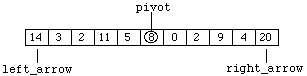Figure 8.7.3

so that "left_arrow" and "right_arrow" initially represent the lowest and highest indices of the array components. Starting on the right, the "right_arrow" is moved left until a value less than or equal to the pivot is encountered. This produces: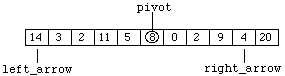Figure 8.7.4

In a similar manner, "left_arrow" is moved right until a value greater than or equal to the pivot is encountered. This is already the situation in our example. Now the contents of the two array components are swapped to produce: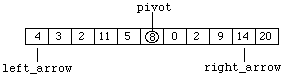Figure 8.7.5

We continue by moving "right_arrow" left to produce: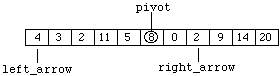Figure 8.7.6

and then "left_arrow" right to produce: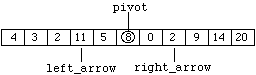Figure 8.7.7

These values are exchanged to produce: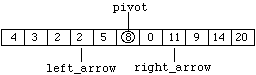Figure 8.7.8

This part of the process only stops when the condition "left_arrow > right_arrow" becomes True. Since in Figure 8.7.8 this condition is still False, we move "right_arrow" left again to produce: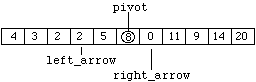Figure 8.7.9

and "left_arrow" right again to produce: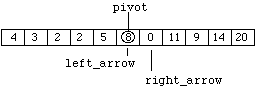Figure 8.7.10

Because we are looking for a value greater than or equal to "pivot" when moving right, "left_arrow" stops moving and an exchange is made (this time involving the pivot) to produce: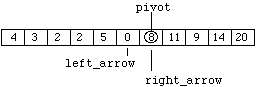Figure 8.7.11

It is acceptable to exchange the pivot because "pivot" is the value itself, not the index. As before, "right_arrow" is moved left and "left_arrow" is moved right to produce: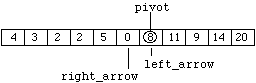Figure 8.7.12

The procedure's terminating condition "left_arrow > right_arrow" is now True, and the first sub-division of the list (i.e. array) is now complete.

Here is the procedure Quick Sort coded up as a C++ function:

```	void quick_sort(int list[], int left, int right)
{
int pivot, left_arrow, right_arrow;

left_arrow = left;
right_arrow = right;
pivot = list[(left + right)/2];

do
{
while (list[right_arrow] > pivot)
right_arrow--;
while (list[left_arrow] < pivot)
left_arrow++;
if (left_arrow <= right_arrow)
{
swap(list[left_arrow], list[right_arrow]);
left_arrow++;
right_arrow--;
}
}
while (right_arrow >= left_arrow);

if (left < right_arrow)
quick_sort(list, left, right_arrow);
if (left_arrow < right)
quick_sort(list, left_arrow, right);
}
```

Fragment of Program 8.7.1

## 8.8 Summary

We have seen that C++ functions may be defined recursively, and have briefly discussed how C++ executes recursively defined functions in terms of the stack. We have discussed the correspondence and relative advantages and disadvantages of recursive function definitions compared with iterative definitions. We have also described the standard sorting algorithm Quick Sort, and shown how it may be implemented in C++ using a recursive function definition. Most of the material here is also covered in more detail in Savitch, Chapter 14.